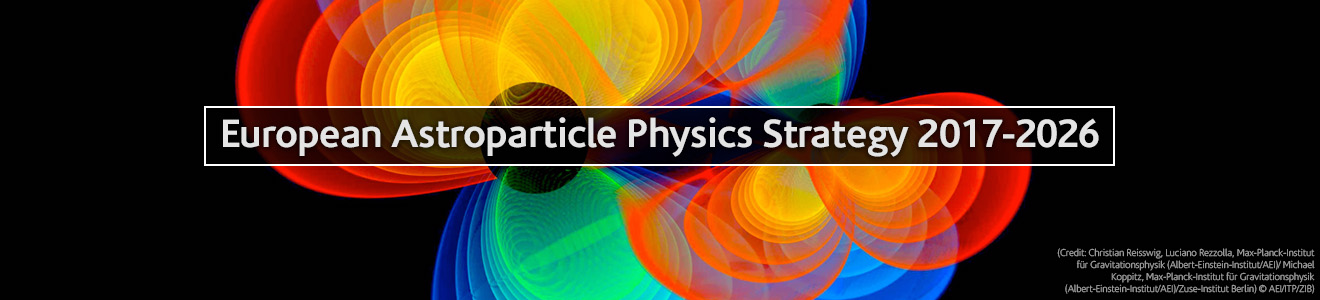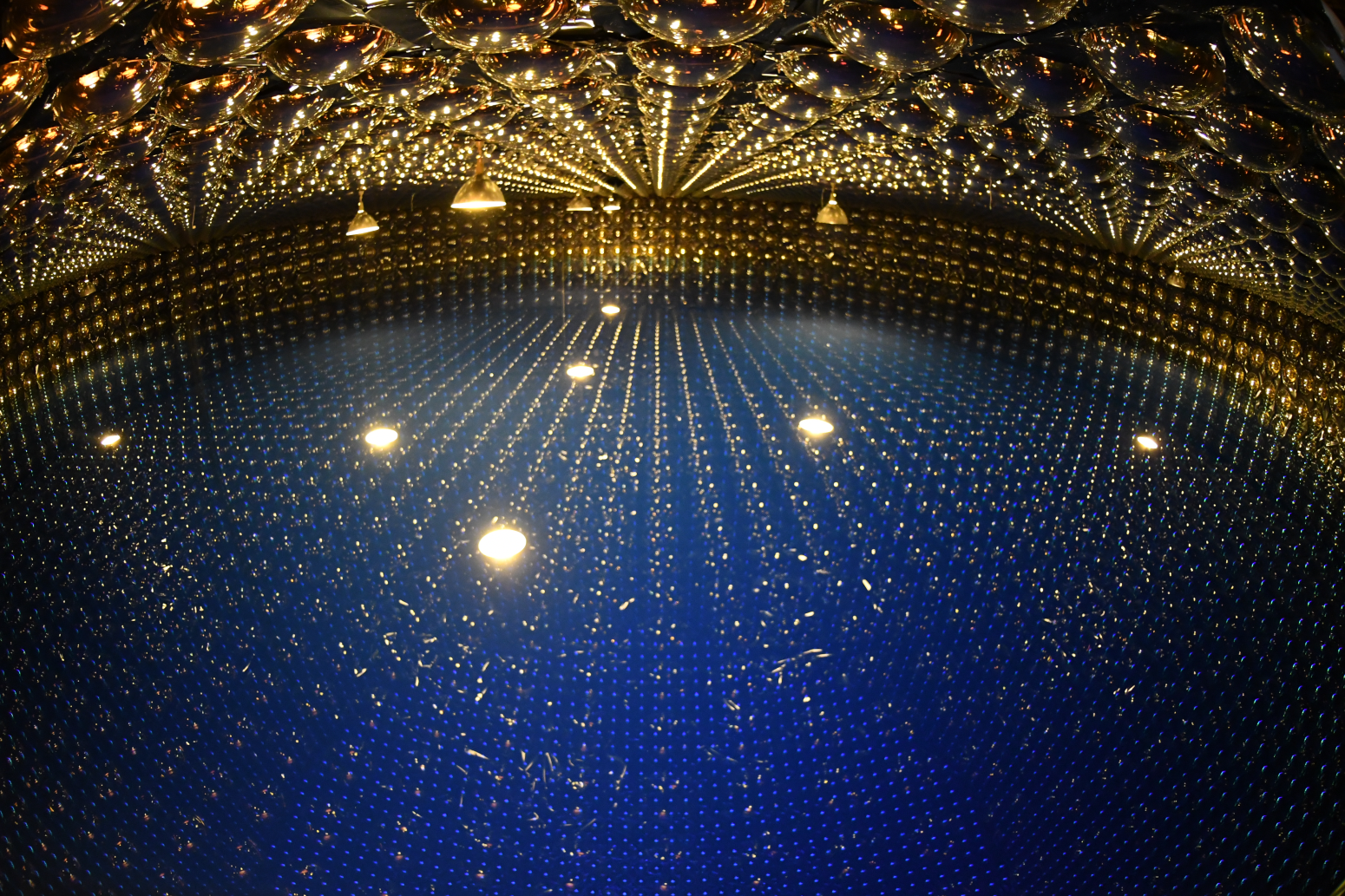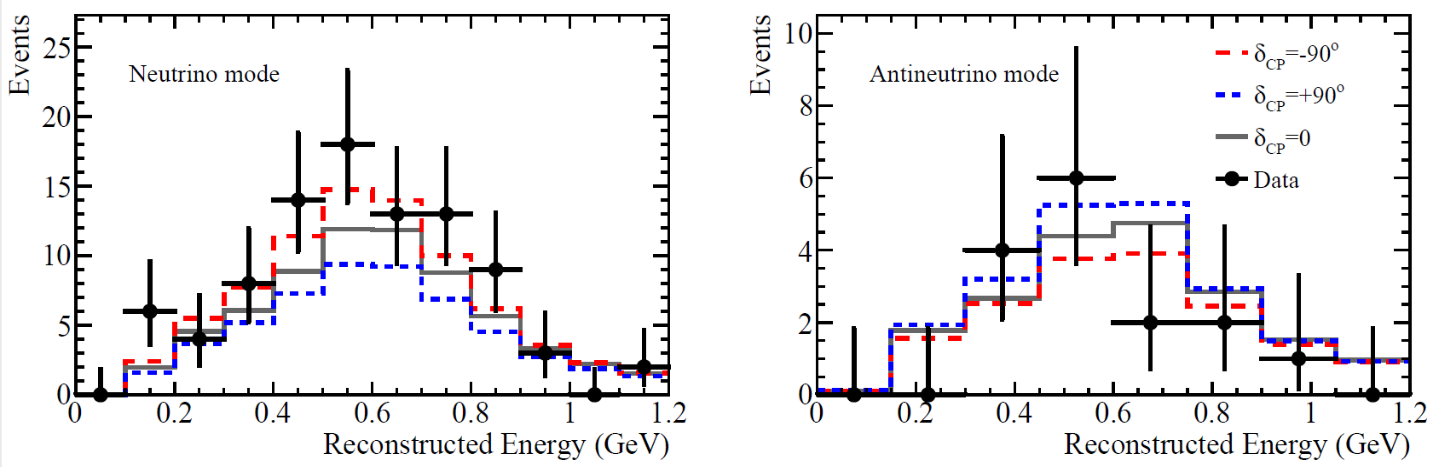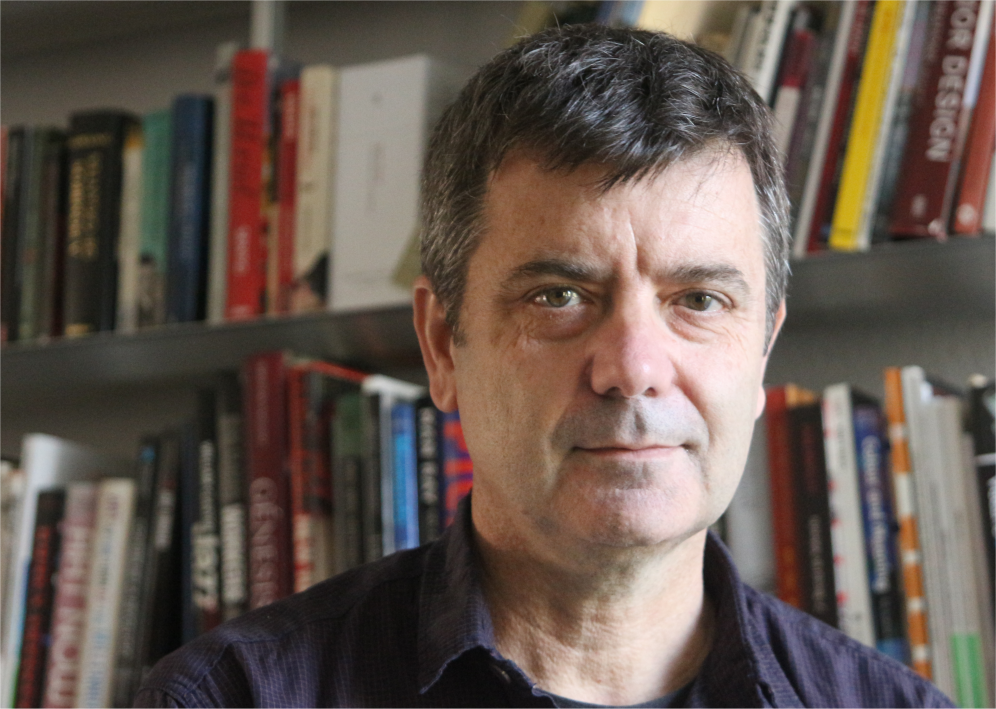•# T2K results constrain possible values of the leptonic CP-violating phase

## Interview with Federico Sanchez about the recent results of T2K collaboration

Recently the T2K experiment published in Nature their results on the constraint of leptonic CP violation. Although there is no one-to-one link between the matter antimatter asymmetry and the value of delta from the T2K measurement, these results are a major step forward in the study of difference between matter and antimatter. Federico Sanchez explains how T2K measures CP violation and what they can conclude.

### Congratulations for your results and their publication. Can you explain why a different behaviour of matter and antimatter is so important?Inside the Super-K detector. Credit: Kamioka Observatory,  Institute for Cosmic Ray Research, University of Tokyo

The different behavior of particle and antiparticles, or matter and antimatter, is by its own a breakthrough result. The different behavior of particle and antiparticles is a possibility contemplated in the Standard Model describing the fundamental particles.  CP violation with leptons is described by a fundamental parameter, the phase angle δCP which is the parameter measured at the T2K experiment. There is no specific prediction of the value of this angle in our theoretical models. Its determination is important to advance in the understanding of the standard model. CP violation is related to flavor-changing mechanisms in the standard model, its measurement may help to understand more deeply the flavor dynamics. Flavor is what physicists identify with the differences between the three lepton families (electron, muon, and tau) or the three quark families. CP violation is a known phenomenon in processes involving quarks since the 1960’s. It has taken the particle physics community almost 60 years to start seen similar behavior in leptons.  I believe this is the most relevant implication of the T2K result.

Besides the relevance to particle physics, CP violation might have implications in the understanding of our matter-dominated Universe. The existence of CP violation mechanism is one of the three conditions proposed by Andrei Sakharov to explain the baryon(or matter) asymmetry of the Universe. Baryon number violation and interactions out of thermal equilibrium are the other two. CP violation is then a necessary condition although not a sufficient one. The CP violation amount and its origin are relevant to model this asymmetry. I would like to stress that we are still far from understanding this mechanism, the baryon number violation has not been proved experimentally so far, and it is not obvious that the CP violation in neutrinos and quarks are the mechanisms required to explain the baryon asymmetry in the universe.  Although some theoretical models connect both phenomena, there is a long way to go. Hopefully, the new results can help in this challenging enterprise.

### Can you explain the measurement principles of T2K?The observed electron neutrino (left) and electron antineutrino (right) candidate events with predictions for maximal neutrino enhancement (red, long dash) and maximum antineutrino enhancement (blue, short dash). Credit: the T2K experiment

T2K collaboration studies the so-called neutrino oscillations. The neutrino oscillation is a quantum mechanical interference caused by the fact that every neutrino of the type electron, muon, or tau is a combination of three neutrino masses.  The neutrino type electron, muon, or tau is determined by the associated heavy lepton (electron, muon, or tau) in the interaction. The neutrino has three paths to travel from the production to the interaction points. Each one associated with one neutrino mass. The neutrinos travel as a superposition of these three states, each one with a different mass and speed, producing the interference patterns. Experimentally, this quantum mechanical interference is measured by looking at the appearance of types of neutrinos at the interaction point different from the ones that were produced. Particularly in T2K, we look for the transformation of muon neutrinos into electron neutrinos. The CP phase induces differences in the oscillation for the neutrinos and its antiparticles, the antineutrinos. In T2K, we have measured the oscillation parameters for neutrinos and antineutrinos and from the difference, we can infer the value of the CP violation phase.  The T2K experiment can produce both neutrinos and antineutrinos simply by focusing or defocusing positively charged pions and negatively charged pions. The positive pions produce neutrinos during its disintegration and negative pions produce antineutrinos.

### Your experiment is sensitive to the δCP Phase, which parameter space can you exclude and what does this mean?The arrow indicates the value most compatible with the data. The gray region is disfavored at 99.7% (3σ) confidence level. Nearly half of the possible values are excluded. Credit: the T2K experiment

The result from T2K excludes half of the possible values of δCP, particularly the positive values of the phase angle are excluded with a confidence level of 99.7%. If we take possible values of δCP from -180 degrees to 180 degrees we excluded values from  -1.7 degrees to 164.6 degrees. This is the first time we have measured experimentally this fundamental parameter in the Standard Model.The other important read of the T2K results is that the most probable value of the δCP is close to -90 degrees implying the maximal violation of the CP symmetry in neutrinos. The fact that it can be maximal open possible ways to understand the mechanism that differentiates neutrino mass states from flavor states.

### What are the consequences of the constrain of T2K on the δCP in the neutrino sector on the matter-anti-matter asymmetry?

When confirmed, the result might have several implications. First of all, it is a new source of CP violation beyond the traditional one in the quark sector. This additional source plus the special properties of neutrinos might explain through a relevant theory the origin of the matter-dominated Universe through theoretical models.  Another relevant implication is related to the value of δCP. If the result is confirmed to be maximal as suggested by T2K, this might have theoretical implications since it might be a reflection of hidden symmetries in a model.

### What are your ideas to further improve the measurements?

In particle physics, 99.7% is not sufficient to claim a discovery. We need values of the confidence level of 99.9999%. To reach this precision we need more data, the 115 events collected by T2K are not enough. To achieve larger statistics there are few venues we are taking. The first one implies running longer time, the second to increase the flux of neutrinos, and third to increase the mass of the far detector.  The first step is just time and money, we will keep running a few years more hopefully doubling or tripling the number of neutrinos we detect. The second step can be done by increasing the total number of protons we can accumulate in the accelerator per unit of time.  Protons produce the pions that subsequently produce neutrinos by decay.  There is already an approved project that will almost double the number of protons during the next years. The third one requires new detectors. Recently, the upgrade of the T2K far detector, SuperKamiokande, was approved by the Japanese authorities. The new project, HyperKamiokande, will increase the detector mass and the number of detected neutrinos per unit of proton in the accelerator by almost a factor of ten.  With this increase, we can accumulate ten times more neutrinos for the same number of protons than we do today.  Unfortunately, this will not be sufficient. In parallel, we need to understand some of the uncertainties of the experiment. These uncertainties are related to better control of the neutrino flux predictions and the modeling of neutrinos interacting with nuclei. Both are at the moment the most relevant non-statistical uncertainties in the measurement and they will become dominant when we increase the number of detected neutrinos. To address these issues, we need supporting experiments to help to understand the production of pions by proton interactions and to improve the understanding of neutrino interactions. We also need to develop more precise theoretical models describing the interaction of neutrinos with nuclei so we can interpret these experiments correctly, and in parallel, we need to prove experimentally they are correct.

#### We would like to add a short comment by Silvia Pascoli in which she discusses the results of the T2K experiment in a theoretical context. We asked her about the connection between T2K results and the baryon asymmetry of the Universe.

A simple assumption, justified by cosmological inflation, is that the Universe at the very beginning contained the same amounts of matter and antimatter. In the 60’ A. Sakharov identified the conditions which are required for some process in the Early Universe to generate a small asymmetry between matter and antimatter: the violation of the C and CP symmetry, lepton (or baryon) number violation, which is testable in neutrino less double beta decay, and the out of equilibrium condition.
Leptogenesis, using leptonic CP violation, is among the favourite explanations of the baryon asymmetry as it takes place in models which have been proposed to explain the observed neutrino masses. Under certain conditions, specifically in see-saw type I neutrino mass models, it has been shown that the leptonic CP violating delta phase searched for in long baseline neutrino oscillation experiments can be the source of the observed matter-antimatter asymmetry. This is a highly non-trivial statement as in many other models the baryon asymmetry that can be generated is too small.
Observing leptonic CP violation and the violation of lepton number would provide circumstantial evidence (although not a proof) towards leptogenesis as the origin of the matter-antimatter asymmetry of the Universe.

#### We asked her to further comment on the connection to neutrinoless double beta decay.

First of all, as I discussed above, lepton number violation is one of the three key criteria for leptogenesis to explain the baryon asymmetry of the Universe. Neutrino less double beta decay is the most sensitive test we have of this global symmetry of the Standard Model. Moreover, the results of T2K and NOvA and other neutrino oscillation experiments on the ordering of neutrino masses play a key role in the predictions for the lifetime of the decay process. So, mass ordering information is very important to plan the future program in this field and to interpret the results from future experiments.